# 常规解法

## 解法二

303 题 做过 sumRange 的优化，我们用一个数组保存累计的和，numsAccumulate[i] 存储 0i - 1 累计的和。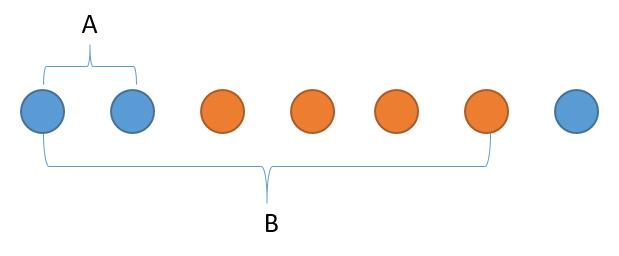# 树状数组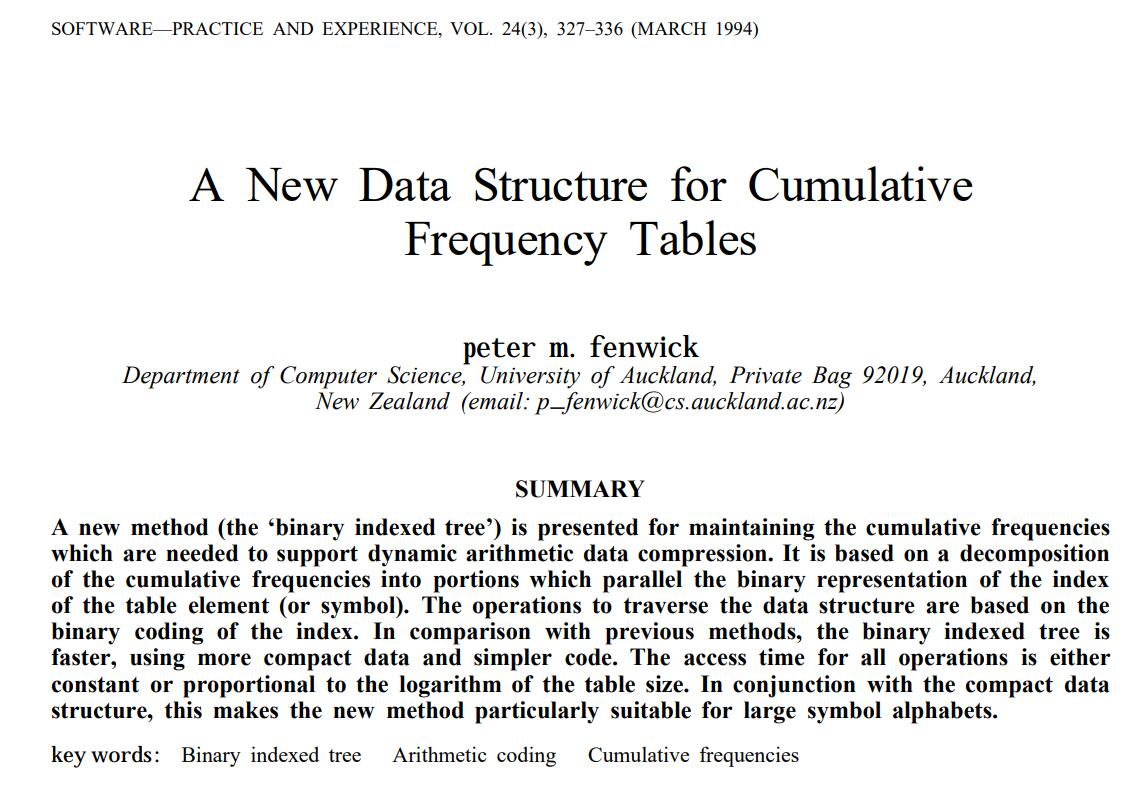[1, x] = [x, x - a + 1] + [x - a, x - a - b + 1] + [x - a - b, x - a - b - c + 1] + ...

[1, 11] = [11, 11] + [10, 9] + [8, 1]

[11, 11][10, 9][8, 1] 长度分别是 128

[1, 11] = [11, 11] + [10, 9] + [8, 1] 我们用 V 表示子区间，用 F 表示某个区间。

F[1,11] = V + V + V

1010 = 1011 - 00010001 就是十进制的 1，所以 V1 个数，所以 V = F[11,11]

1000 = 1010 - 00100010 就是十进制的 2，所以 V2 个数，所以 V = F[10,9]

0000 = 1000 - 10001000 就是十进制的 8，所以 V8 个数，所以 V = F[8...1]

V1 个数， V2 个数，看的是二进制最右边的一个 1 到末尾的大小。1010 就是 101000 就是 1000

F[1,11] = BIT + BIT + BIT，看下二进制 BIT[0001,1011] = BIT + BIT + BIT

1011 -> 1010 -> 1000，对于 BIT 每次的下标就是依次把当前数最右边的 1 变成 0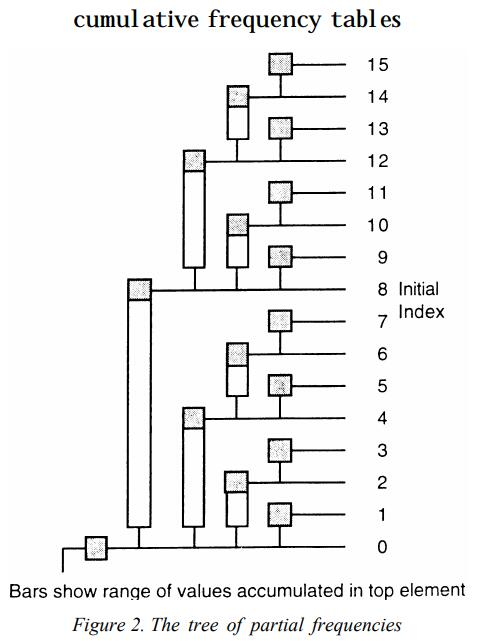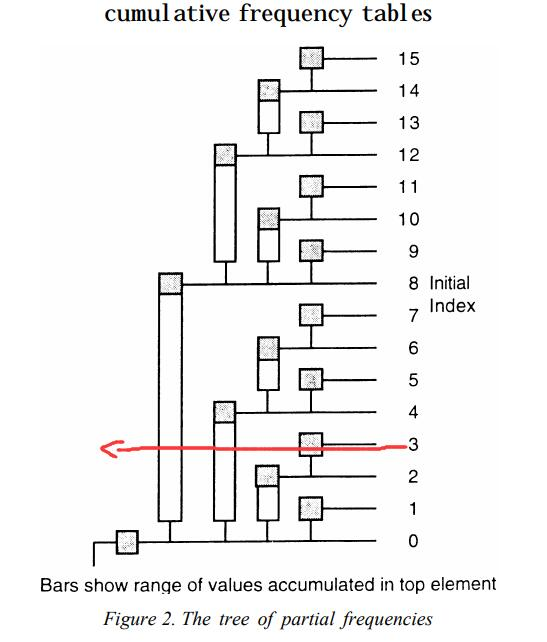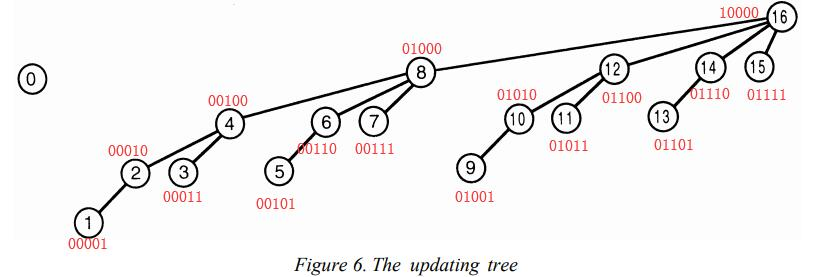00101 -> 00110 -> 01000 -> 10000

00101 + 1 = 00110

00110 + 10 = 01000

01000 + 1000 = 10000

# 总